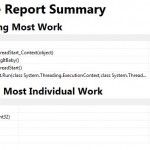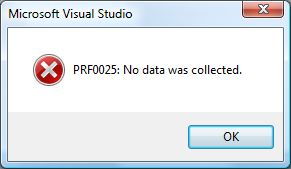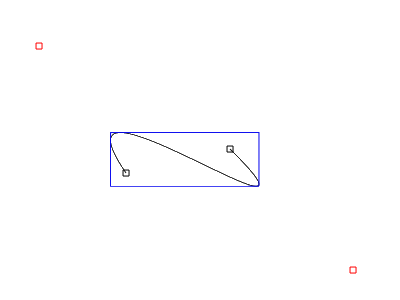## String equals based on Regex C#

I need to compare strings based on what is matched by a regex. So I decided to create a regex to pick the parts of a string that I felt was necessary for consideration for equals. When using a regex and leaving parts of the string outside use “non capturing groups”.   Like “(\d+)(?:st|nd|rd|th)?” with will match a number and optional some postfix like “nd” as in 2nd.

```public static class StringEqualsRegex
{
/// <summary>
/// Equals the strings by regex.
/// </summary>
/// <param name="s1">The first string.</param>
/// <param name="s2">The second string.</param>
/// <param name="regex">The regex.</param>
/// <returns><c>true</c> if the captured strings of s1 and s2 match, <c>false</c> otherwise.</returns>
public static bool EqualsByRegex(this string s1, string s2, Regex regex)
{
/*Extension Method*/
return EqualStringByRegex(s1, s2, regex);
}

/// <summary>
/// Equals the strings by regex.
/// </summary>
/// <param name="s1">The first string.</param>
/// <param name="s2">The second string.</param>
/// <param name="regex">The regex.</param>
/// <returns><c>true</c> if the captured strings of s1 and s2 match, <c>false</c> otherwise.</returns>
public static bool EqualStringByRegex(string s1, string s2, Regex regex)
{

string capturedS1 = GetCapturedString(s1, regex);
string capturedS2 = GetCapturedString(s2, regex);

return (capturedS1 != null && capturedS2 != null) &&
capturedS1.Equals(capturedS2);
}

private static string GetCapturedString(string s, Regex regex)
{
dynamic match = regex.Match(s);

if ((match.Success))
{
var sb = new StringBuilder();
for (var i = 1; i <= match.Groups.Count; i++)
{
foreach (Capture capture in match.Groups[i].Captures)
{
sb.Append(capture.Value);
}
}
return sb.ToString();
}

return null;
}

}
```

.

[wpdm_file id=1]

## Type.GetType, partial assembly names. Getting types of an assambly in the GAC.

Consider this piece of code of a console application. (System.Drawing) is NOT referenced!

```            //Find by partial Name.
Type partial = Type.GetType("System.Drawing.Rectangle, System.Drawing");
//Find type with Assambly identity
Type byIdent = Type.GetType("System.Drawing.Rectangle, System.Drawing, version=2.0.0.0,publicKeyToken=b03f5f7f11d50a3a,culture=neutral");

if (partial == null)
Console.WriteLine("alter app.config to let this work");

if (byIdent == null)

There are 2 way’s to load a type with partial assambly name.
1) place the assambly in the executing directory, the file will be found. (Consider the installation implications!)

```<?xml version="1.0" encoding="utf-8" ?>
<configuration>
<runtime>
<assemblyBinding xmlns="urn:schemas-microsoft-com:asm.v1">
<qualifyAssembly partialName="System.Drawing" fullName="System.Drawing,version=2.0.0.0,publicKeyToken=b03f5f7f11d50a3a,culture=neutral"/>
</assemblyBinding>
</runtime>
</configuration>```

## Profiling a Windows Service

You need to do a little bit extra to profile a service, if you get “VSPerfCLREnv was not set up correctly” solution is here.

This “howto” has been written for a really simple service, wich you can download. It’s tiny so you can isolate the “how do i” from the “for my service”.

To quickly start do this:

run “VSPerfCLREnv.cmd /globalsampleon” this cmd is located in “Team Tools\Performance Tools”(Vs2008/vs2010) install folder, for me that is : “C:\Program Files\Microsoft Visual Studio 9.0\Team Tools\Performance Tools\” . This will be “C:\Program Files\Microsoft Visual Studio 10.0\Team Tools\Performance Tools\” for vs2010.

Open your “windows service” project in Visual Studio.

When you are using my sample do this:

Compile solution (this installs the service and starts the service, see: “build event”)

Start Wizzard : Analyze > Launch Performance Wizzard , select “sampling” and finish.

Now you can’t “Launch with Profiling” (you can’t start a service directly), you need to “Attach” to it, find your service process. Check the “Show processes from all users” and “Show processes in all sessions“.

Run it as long as you wish until you are done. Press stop and you’ll receive report which you can drill down into.Now in real live applications your service might not be a hog for cpu cycles. So you might get an error that “No Data was Collected“.This could happen:

• You did not attach to correct process!

Finally:

When you are done with profiling call “VSPerfCLREnv.cmd” /globaloff” this will disable profiling and give you back those cpu cycles otherwise used for profiling.

## Calculating / Computing the Bounding Box of Cubic Bezier

Goal: Showing an algo that computes the Bounding box of a cubic bezier.
Audience: Needs some basic Math, Derivative, solving parabola, knowledge of computer language.
Sample in c# : coming soon, at bottom of article.

This is also my first usage of a HTML5 element, so IE users sorry!

If you are NOT familiar with what a cubic bezier is, please look at this wiki page.

If you use the start and end point of a cubic bezier and both of the controlpoints, you will end up with a box. This box will contain the bezier, but it’s not really tight. You want a tight bb (bounding box). The controlpoints of the bezier may lay outside the bb.

This example is shows a working example (if the HTML5 canvas element is supported you’ll see a interactive demonstration) :

Sorry the CANVAS tag is not supported by your browser (are you browsing with IE??) 🙁So how to do this?

You’ll need to know the function of the cubic bezier. Defined as:
f(t) = a*t^3 + b*t^2 + c*t +d
where
d = P0
c = 3*P1-3*P0
b = 3*P2-6*P1+3*P0
a = P3-3*P2+3*P1-P0

p0 and p3 are the begin and end point of the bezier, p1 & p2 the control points. This function is defined for t=[0..1] so (0, 0.00001, 0.00002, … 0.99999)

Code to draw bezier point by point

```
protected override void OnPaint(PaintEventArgs pe)
{
Func<double, double, double, double, double, double> bezierSpline = (p0, p1, p2, p3, t) =>
(p3 - 3 * p2 + 3 * p1 - p0) * Math.Pow(t, 3)
+ (3 * p2 - 6 * p1 + 3 * p0) * Math.Pow(t, 2)
+ (3 * p1 - 3 * p0) * t
+ (p0);

for (double t = 0; t <= 1; t += 0.001) //adjust if you want
{
double x = bezierSpline(_points.X, _points.X, _points.X, _points.X, t);
double y = bezierSpline(_points.Y, _points.Y, _points.Y, _points.Y, t); ;

var p = new PointF((float)x, (float)y);
drawPoint(pe.Graphics, p, Color.Orange, 2);
}
}

private void drawPoint(Graphics g, PointF p, Color color, float size)
{
Brush b = new SolidBrush(color);
float w = size;
RectangleF r = new RectangleF(p.X-(w/2f),p.Y-(w/2f),w,w);
g.FillEllipse(b,r);
b.Dispose();
}//this code snippet has been cut-n-pasted from project, may contain some err..
```

A possible way to compute bb is to add each point to bb evaluation, and you need plenty to ensure the exactness of the bb! Don't do this! Unless you want to do TDD and test if the bb is nearly the same as the bb you are about to compute for testing purposes.

### Derivative

The exact solution can be found by using math. if you derivative the bezier function you can find local extremes.

so
f(t) = a*t3 + b*t2 + c*t +d
becomes :
f(t)' = 3a*t^2 + 2b*t + c

Do not forget the values of a,b and c! as defined above.

If you find the where f(t)'=0 then you'll find local extremes. To do this you'll need to find where the parabola equals 0 for each of the axises. You'll need to solve the parabola (f(t)' is now a parabola!) for the x and y values of your points.

To find out if there is a solution we'll use the discriminant (Quadratic formula) b^2-4ac. This will tell you if there is a solution.

Example:
suppose points:
p0 = (30,70)
p1 = (0,270)
p2 = (290,110)
p3 = (200, 100)

f(t)' = 3a*t^2 + 2b*t + c

with:

a = P3-3*P2+3*P1-P0
b = 3*P2-6*P1+3*P0
c = 3*P1-3*P0

becomes:

f(t)' = 3(P3-3*P2+3*P1-P0)*t^2 + 2(3*P2-6*P1+3*P0)*t + (3*P1-3*P0)

//solution for x values:
a = 3(200-3*290+3*0-30) = -2100
b = 2(3*P2-6*P1+3*P0) = 1920
c = (3*P1-3*P0) = -90

with D = b^2-4ac
D=2930400
D>0 so there are 2 solutions

you can calculate the solution with (-b + sqrt(b^2 - 4ac)) / 2a and (-b - sqrt(b^2 - 4ac)) / 2a.

s1 = 0.04956 s2 = 0.86472

do the same for the Y values
you'll come to:
a=1530
b=-2160
c=600

D=993600 so 2 solutions

s1=1.03163
s2=0.38013

!!You'll need to throw away the first solution of 1.03163, since the bezier function is only defined between 0 and 1!

us the solutions you came up as params for the original bezier function.
so :
//s1 = 0.04956 s2 = 0.86472
x1 = f(0.04956) => 27.8
y1 = f(0.04956) => 97.1

x2 = f(0.86472) => 217.3
y2 = f(0.86472) => 111.0

//s1=1.03163 s2=0.38013
x3 = f(0.38013) => 96.0
y3 = f(0.38013) => 170.0

ok,.. now you have the local extremes! And evaluation of the bb is next.

### The Bounding Box

Take the start and end point, include the local extremes.

find the smallest x and y value
find the largest x and y value.

Now there is a small trap,..
your p0..p3 can be placed in such a way that for the f(t)' a becomes 0. When this happens the function is no longer a parabola, but a line.
f(t)' = at^2 + bt + c
f(t)' = at^2 + bt + c
f(t)' = bt + c
-bt= c
-t = c/b
t = -c/b

that is all you'll need to solve the bb of a bezier!

## C# saving as EMF

This is a useful piece of code, created as an extension method on MetaFile to save a Metafile as a real Vector based emf not as a PNG. See : msdn on image encoders / decoders.

Here is the code:

```using System;
using System.Collections.Generic;
using System.Linq;
using System.Text;
using System.Runtime.InteropServices;
using System.IO;

namespace System.Drawing.Imaging
{
public static class ExtensionMethods
{
public static void SaveAsEmf(this Metafile me, string fileName)
{
How to save or serialize a Metafile: Solution found
by : SWAT Team member _1
Date : Friday, February 01, 2008 1:38 PM
*/
int enfMetafileHandle = me.GetHenhmetafile().ToInt32();
int bufferSize = GetEnhMetaFileBits(enfMetafileHandle, 0, null); // Get required buffer size.
byte[] buffer = new byte[bufferSize]; // Allocate sufficient buffer
if (GetEnhMetaFileBits(enfMetafileHandle, bufferSize, buffer) <= 0) // Get raw metafile data.
throw new SystemException("Fail");

FileStream ms = File.Open(fileName, FileMode.Create);
ms.Write(buffer, 0, bufferSize);
ms.Close();
ms.Dispose();
if (!DeleteEnhMetaFile(enfMetafileHandle)) //free handle
throw new SystemException("Fail Free");
}

[DllImport("gdi32")]
public static extern int GetEnhMetaFileBits(int hemf, int cbBuffer, byte[] lpbBuffer);

[DllImport("gdi32")]
public static extern bool DeleteEnhMetaFile(int hemfbitHandle);
}
}
```

How to use this code?

```            //Set up reference Graphic
Graphics refG = this.CreateGraphics(); //assumin this code is running on a control/form
IntPtr refGrap = refG.GetHdc();
var img = new Metafile(refGrap, EmfType.EmfPlusDual, "..");

//Draw some silly drawing
using (var g = Graphics.FromImage(img))
{
var r = new Rectangle(0,0,100,100);
var reye1 = new Rectangle(20, 20, 20, 30);
var reye2 = new Rectangle(70, 20, 20, 30);

var pBlack = new Pen(Color.Black, 3);
var pRed = new Pen(Color.Red, 2.5f);

g.FillEllipse(Brushes.Yellow, r);
g.FillEllipse(Brushes.White, reye1);
g.FillEllipse(Brushes.White, reye2);
g.DrawEllipse(pBlack, reye1);
g.DrawEllipse(pBlack, reye2);
g.DrawBezier(pRed, new Point(10, 50), new Point(10, 100), new Point(90, 100), new Point(90, 50));
}

refG.ReleaseHdc(refGrap); //cleanup
refG.Dispose();

img.SaveAsEmf("test.emf");  //chose this line

//img.Save("test2.emf", ImageFormat.Emf); //or this line
```

The end result:Tags
Categories
Meta